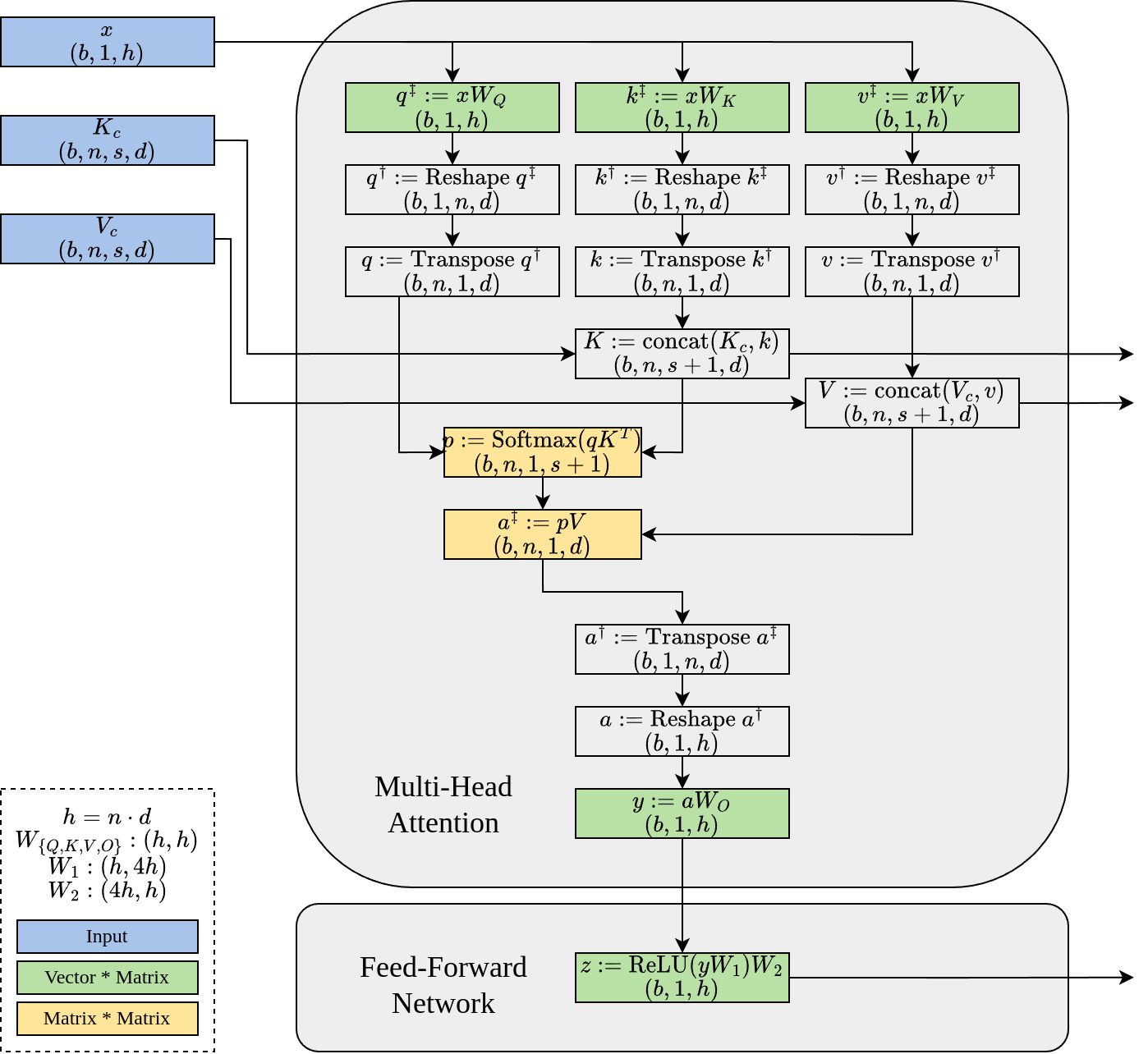## 背景知识：文本生成

• 预填充阶段（或称“编码”，“初始化”）接受整个提示并生成随后的词元以及一个“键值缓存”(KV Cache)。
• 解码阶段处理新生成的词元和键值缓存，然后生成下一个词元，同时更新键值缓存。这个阶段不断重复，直到模型完成其输出。

## 背景知识：大语言模型的架构和批处理• 自注意力（Self-Attention，黄色标出）涉及矩阵-矩阵乘法。
• 密集投影（Dense Projection，绿色标出）涉及向量-矩阵乘法。

## LoRA 延迟和批处理效应

h1 = 4096
h2 = 11008
r = 16
for bs in range(1, 33):
w = torch.randn(h1, h2, dtype=torch.float16, device="cuda:0")
a = torch.randn(bs, h1, r, dtype=torch.float16, device="cuda:0")
b = torch.randn(bs, r, h2, dtype=torch.float16, device="cuda:0")
x = torch.randn(bs, 1, h1, dtype=torch.float16, device="cuda:0")
bench(lambda: x @ w)
bench(lambda: x @ a @ b)$\begin{pmatrix} \vec{y_1} \\ \vec{y_2} \\ \vdots \\ \vec{y_b} \end{pmatrix} := \begin{pmatrix} \vec{x_1} \\ \vec{x_2} \\ \vdots \\ \vec{x_b} \end{pmatrix} W + \begin{pmatrix} \vec{x_1}A_1B_1 \\ \vec{x_2}A_2B_2 \\ \vdots \\ \vec{x_b}A_bB_b\end{pmatrix}$

## 批处理 LoRA 算子

def add_lora(
y: torch.Tensor,      # (batch_size, 1, out_features)
x: torch.Tensor,      # (batch_size, 1, in_features)
A: torch.Tensor,      # (num_loras, in_features, lora_rank)
B: torch.Tensor,      # (num_loras, lora_rank, out_features)
I: torch.LongTensor,  # (batch_size,)
):
"""Semantics: y[i] += x[i] @ A[I[i]] @ B[I[i]]"""
raise NotImplementedError()


def lora_loop(
y: torch.Tensor,  # (batch_size, 1, out_features)
x: torch.Tensor,  # (batch_size, 1, in_features)
A: torch.Tensor,  # (num_loras, in_features, lora_rank)
B: torch.Tensor,  # (num_loras, lora_rank, out_features)
I: torch.LongTensor,  # (batch_size,)
):
for i, idx in enumerate(I.cpu().numpy()):
y[i] += x[i] @ A[idx] @ B[idx]


def lora_cheat_bmm(
y: torch.Tensor,  # (batch_size, 1, out_features)
x: torch.Tensor,  # (batch_size, 1, in_features)
cheat_A: torch.Tensor,  # (batch_size, in_features, lora_rank)
cheat_B: torch.Tensor,  # (batch_size, lora_rank, out_features)
):
y += x @ cheat_A @ cheat_B

num_loras = 50
h1 = 4096
h2 = 11008
r = 16
A = torch.randn(num_loras, h1, r, dtype=torch.float16, device="cuda:0")
B = torch.randn(num_loras, r, h2, dtype=torch.float16, device="cuda:0")
for bs in range(1, 33):
x = torch.randn(bs, 1, h1, dtype=torch.float16, device="cuda:0")
y = torch.randn(bs, 1, h2, dtype=torch.float16, device="cuda:0")
I = torch.randint(num_loras, (bs,), dtype=torch.long, device="cuda:0")
cheat_A = A[I, :, :]
cheat_B = B[I, :, :]

bench(lambda: lora_loop(y, x, A, B, I))
bench(lambda: lora_cheat_bmm(y, x, cheat_A, cheat_B))def lora_gbmm(
y: torch.Tensor,  # (batch_size, 1, out_features)
x: torch.Tensor,  # (batch_size, 1, in_features)
A: torch.Tensor,  # (num_loras, in_features, lora_rank)
B: torch.Tensor,  # (num_loras, lora_rank, out_features)
I: torch.LongTensor,  # (batch_size,)
):
a = torch.index_select(A, 0, I) # (batch_size, in_features, lora_rank)
b = torch.index_select(B, 0, I) # (batch_size, lora_rank, out_features)
y += x @ a @ b


## BGMV 算子def lora_bgmv(
y: torch.Tensor,  # (batch_size, 1, out_features)
x: torch.Tensor,  # (batch_size, 1, in_features)
A: torch.Tensor,  # (num_loras, in_features, lora_rank)
B: torch.Tensor,  # (num_loras, lora_rank, out_features)
I: torch.LongTensor,  # (batch_size,)
):
tmp = torch.zeros((x.size(0), A.size(-1)), dtype=x.dtype, device=x.device)
bgmv(tmp, x, A, I)
bgmv(y, tmp, B, I)## 多租户 LoRA 文本生成性能

bgmv算子的基础上，我开发了一个名为Punica的实验项目，支持多个 LoRA 模型。 Punica 的独特能力是能把不同 LoRA 模型的请求合并在一个批处理中。我对比了 Punica 和一系列知名系统的性能，包括HuggingFace TransformersDeepSpeedFaster TransformervLLMDeepSpeed、vLLM 和 Faster Transformers 都有高度优化的 Transformer 实现，这些系统的吞吐量比标准的 HuggingFace Transformers 高出3倍。但由于批处理大小被限制在1，它们在多租户 LoRA 服务效率方面表现不佳。## 示例用途

• 用一本新的小说对 LoRA 模型进行细调，以帮助读者总结每个角色的旅程。
• 针对迅速发展的新闻对 LoRA 模型进行调整，以让读者了解最新动态。
• 基于网页内容对 LoRA 模型进行优化，提高读者的理解能力。